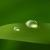# 谁来详细帮我解下这3个题。。万分感谢。

1.

MaxSum = 0;
for (i=0; i<n; i++) {
ThisSum = 0;
for (j=i; j<n; j++) {
ThisSum = ThisSum + A[j];
MaxSum = max(MaxSum,ThisSum);

}

这个代码中ThisSum = ThisSum + A[j]用n表示出这个函数是什么？

2.

Int bar char a[], int s, int t）｛

Ifs>=t,return A;

If(a[s]==a[t]){

Return bar (a, B, C);}

Else{

Return 0;}

｝int foo(char a[]){return bar (a, 0,strlen(a)-1);

a）在插入运算oh）的时间里能处理吗？根据是什么？这里的是双重搜索树的高度

B）最大值删除运算oh）的时间里能处理吗？根据是什么？

0